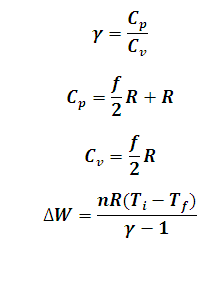# Work Done in Adiabatic Process Calculator

This Calctown Calculator calculates the work done by the system in an Adiabatic Process.

K
K
mol

#### Result

J/K
J/K
Jwhere

ΔW = work done by the system

n = number of moles

R = universal gas constant

Ti = initial temperature

Tf = final temperature

Cp = specific heat at constant pressure

Cv = specific heat at constant volume

f = degree of freedom